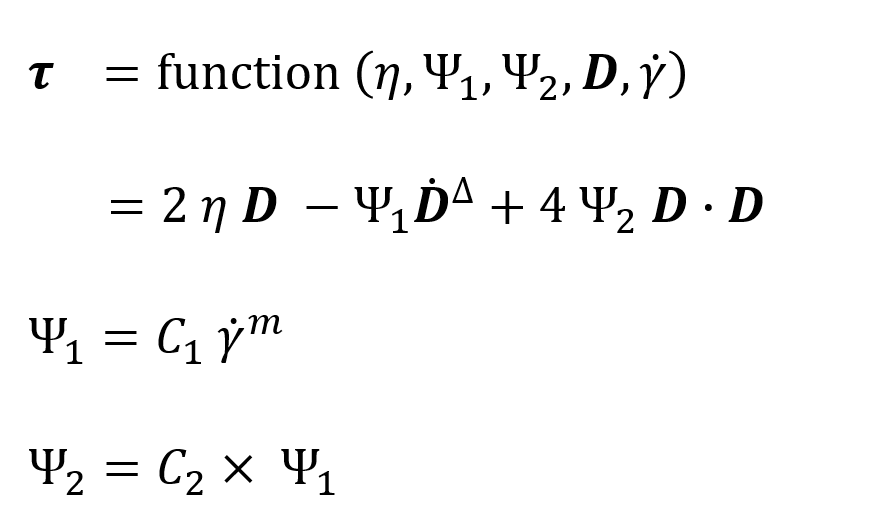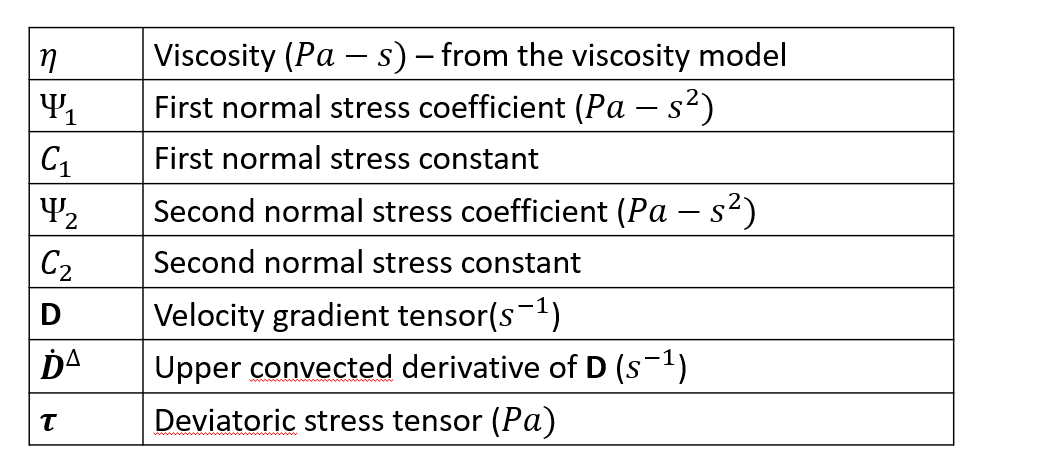# Criminale-Ericksen-Filbey Viscoelastic Model

In this model, the deviatoric part of the stress tensor is expressed as function of the viscosity and the normal stress coefficients.This model can be used with any of the above mentioned non-Newtonian models and the specific model selected is used only to describe the viscosity of the material. The first and second normal stress coefficient functions are described as an exponential function of the computed shear rate. In this specific implementation, the second normal stress coefficient is implemented as a function of the first normal stress coefficient.Parameter Description Units Data Type Typical Value
ViscoElasticModel Two valid choices are “None” (default) and “CEF” None String "CEF"
CEF_Coefficient1 Constant in the exponential function describing the first normal stress coefficient Pa/s2 Constant 1.0e+04
CEF_Coefficeint2 Constant in the exponential function describing the second normal stress coefficient Pa/s2 Constant -.10
CEF_Exponent Exponent in the function describing the first normal stress coefficient None Constant -1.5